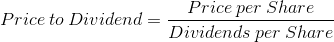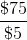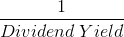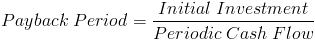Price to Dividend RatioThe price to dividend ratio is calculated by dividing the price per share by the dividends paid per share.

A company's share price, the numerator, can be found through quotes on the secondary stock market. The denominator, dividends per share, can be found on a company's cash flow statement, however many companies that provide stock quotes, will also provide other stock metrics, including the amount of dividends paid per share.

Keep in mind that different stocks pay their dividends at different increments throughout the year. Some company's will pay monthly, some quarterly, et cetera. When comparing metrics among multiple companies, it is important to make equal comparisons. It is best to look at the total annual dividends paid by the company. For example, if one company pays \$1 quarterly per share, and another company pays \$4 per annum, both would be calculated using \$4 in the denominator as this is the annual amount paid for both companies.

Example of the Price to Dividend Ratio

An example of the price to dividend formula would be to assume that the share price of a company's stock is \$75.00. If the dividends that are paid out per share is \$5 per year, we can show this aswhich shows a price to dividend of 15.

Use of the Price to Dividend Ratio

The price to dividend ratio, along with other formulas, can be used by investors when trying to value a stock. Specifically, investors who engage in dividend investing, may find this formula useful.

The price to dividend ratio is the inverse of the dividend yield formula. This is shown asFor example, a 5% dividend yield will have a price to dividend of 20.

In addition to comparing this ratio to dividend yield, it can also be compared to the payback period formula. The payback period formula is used in corporate finance to measure how long it will take for the company to recuperate their initial investment. The formula for payback period isNotice that the time value of money is not taken into consideration here. Also, this comparison is not exactly parallel as the stock could still have a sell price apart from its dividends after the given number of periods. In contrast, the initial investment for a corporate project will be an expense only. Effectively, the price to dividend ratio does show how many years it will take for dividends to yield the original share price. If an investor invests for dividends, they may find this useful.

New to Finance?